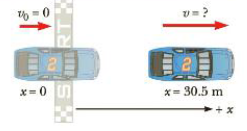Chapter 2, Problem 26P

Chapter
Section
Textbook Problem

PROBLEM A race car starting from rest accelerates at a constant rate of 5.00 m/s2, (a) What is the velocity of the car after it has traveled 1.00 × 102 ft? (b) How much time has elapsed? (c) Calculate the average velocity two different ways.STRATEGY We’ve read the problem, drawn the diagram in Figure 2.16, and chosen a coordinate system (steps 1 and 2). We'd like to find the velocity v after a certain known displacement Δx. The acceleration a is also known, as is the initial velocity v0 (step 3, labeling, is complete), so the third equation in Table 2.4 looks most useful for solving part (a). Given the velocity, the first equation in Table 2.4 can then be used to find the time in part (b). Part (c) requires substitution into Equations 2.2 and 2.7, respectively.Figure 2.16 (Example 2.4)SOLUTION(a) Convert units of Δx to SI, using the information in the inside front cover.Write the kinematics equation for v2 (step 4):Solve for v, taking the positive square root because the car moves to the right (step 5):Substitute v0 = 0, a = 5.00 m/s2, and Δx = 30.5 m:1.00 × 102ft = (1.00 × 102 ft)v2 = v02 + 2a Δxv = v 0 2  + 2 a Δ x v = v 0 2  + 2 a Δ x = ( 0 ) 2  + 2 ( 5.00  m/s 2 ) ( 30.5   m ) = 17.5 m/s(b) Find the trooper's speed at that time. Substitute the time into the trooper’s velocity equation:vtrooper = v0 + atrooper t = 0 + (3.00m/s2)(16.9s)= 50.7 m/sSolve Example 2.5, “Car Chase,” by a graphical method. On the same graph, plot position versus time for the car and the trooper. From the intersection of the two curves, read the time at which the trooper overtakes the car.

To determine
The time at which the trooper overtakes the car.

Explanation

Formula used to find the position of the car is,

xc=x0c+vct

• xc is position of the car at any instant
• x0c is the initial position of the car
• vc is the velocity of car
• t is the time

Substitute 24.0m for x0c and 24.0m/s for vc to find xc .

xc=24.0m+(24.0m/s)t

Formula used to find the position of the trooper is,

xt=12at2

• xt is position of the trooper at any instant
• a is the acceleration of trooper
• t is the time

Substitute 3

Still sussing out bartleby?

Check out a sample textbook solution.

See a sample solution

The Solution to Your Study Problems

Bartleby provides explanations to thousands of textbook problems written by our experts, many with advanced degrees!

Get Started